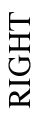### Home > CCG > Chapter 9 > Lesson 9.2.3 > Problem9-91

9-91.

Examine the mat plan of a three-dimensional solid at right.

1. On your paper, draw the front, right, and top views of this solid.

Find the largest number in each column.

For each column, model that number of unit cubes stacked vertically.

Find the right view using the same technique, except going by row instead of column.

Find the top view by noting which squares have at least one unit cube.

2. Find the volume of the solid.

The volume can easily be found by finding the sum of all the numbers on the mat plan, which represents the total number of unit cubes used to construct the solid.

3. If the length of each edge of the solid is divided by $2$, what will the new volume be? Show how you got your answer.

Refer to the Math Notes box from Lesson 9.1.5.

$3\text{ units}^3$

 $6$ $0$ $0$$3$ $0$ $1$ $2$ $6$ $6$ FRONT Mat Plan

Use the eTool below to help solve the problem.
Click the link at right for the full version of the eTool: 9-91 HW eTool